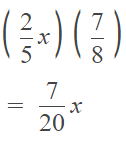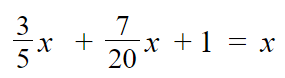# Math in Focus Grade 5 Cumulative Review Chapters 3 and 4 Answer Key

Go through the Math in Focus Grade 5 Workbook Answer Key Cumulative Review Chapters 3 and 4 to finish your assignments.

## Math in Focus Grade 5 Cumulative Review Chapters 3 and 4 Answer Key

Concepts and Skills
Shade and label the model to show the sum of $$\frac{1}{3}$$ and $$\frac{3}{5}$$.
Then complete the addition sentence. (Lesson 3.1)

Question 1.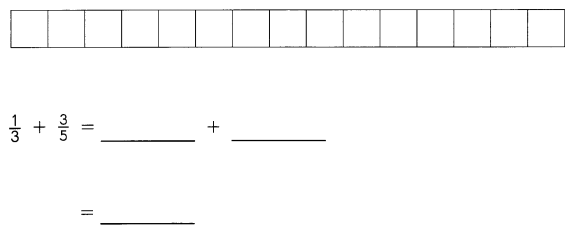$$\frac{1}{3}$$ + $$\frac{3}{5}$$ =
$$\frac{5}{15}$$ + $$\frac{9}{15}$$.=
$$\frac{14}{15}$$
Explanation:
Making unlike denominators to like denominators.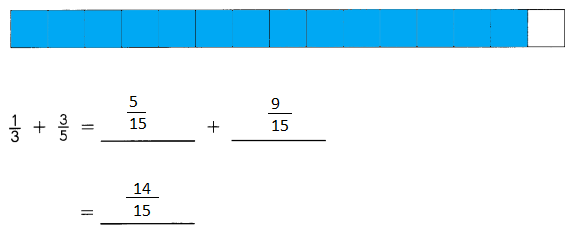Explanation:
Making unlike denominators to like denominators.

Add. Express each sum in simplest form. (Lesson 3.1)

Question 2.
$$\frac{3}{4}$$ + $$\frac{1}{12}$$ =
$$\frac{3}{4}$$ + $$\frac{1}{12}$$ =
$$\frac{9}{12}$$ + $$\frac{1}{12}$$ =
$$\frac{10}{12}$$
Explanation:
Making unlike denominators to like denominators.

Question 3.
$$\frac{3}{5}$$ + $$\frac{2}{7}$$ =
$$\frac{3}{5}$$ + $$\frac{2}{7}$$ =
$$\frac{21}{35}$$ + $$\frac{10}{35}$$ =
$$\frac{31}{35}$$
Explanation:
Making unlike denominators to like denominators.

Estimate each sum by using the benchmarks, 0, $$\frac{1}{2}$$ or 1. (Lesson 3.1)

Question 4.
$$\frac{8}{9}$$ + $$\frac{2}{5}$$ =
= $$\frac{3}{2}$$
Explanation:
Estimating $$\frac{8}{9}$$ as 1
and $$\frac{2}{5}$$  as $$\frac{1}{2}$$
1 + $$\frac{1}{2}$$
= $$\frac{3}{2}$$

Question 5.
$$\frac{1}{8}$$ + $$\frac{6}{7}$$ + $$\frac{1}{6}$$ =
$$\frac{1}{8}$$ + $$\frac{6}{7}$$ + $$\frac{1}{6}$$ =
Explanation:
Estimating the bench marks
$$\frac{1}{8}$$ = 0
$$\frac{6}{7}$$ = 1
$$\frac{1}{6}$$ = 0
0 + 1  + 0 = 1

Shade and label the model to show the difference between $$\frac{4}{5}$$ and $$\frac{2}{3}$$. Then complete the subtraction sentence. (Lesson 3.2)

Question 6.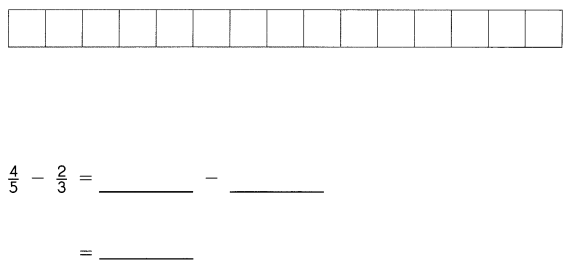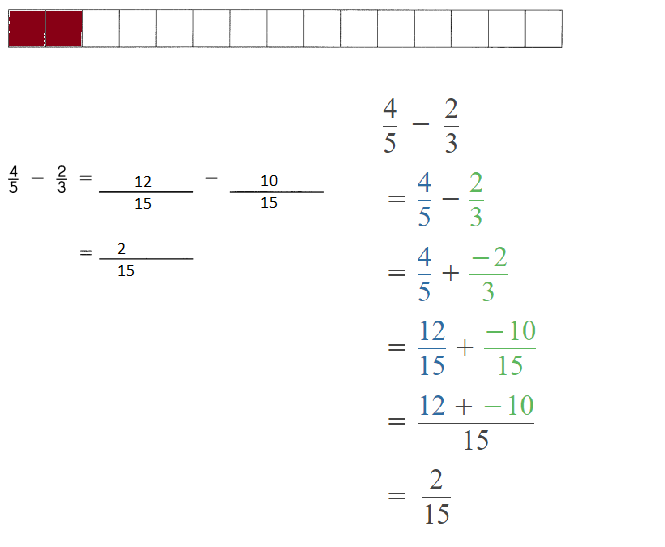Explanation:
Simplified the fractions.

Subtract. Express each difference in simplest form. (Lesson 3.2)

Question 7.
$$\frac{3}{4}$$ – $$\frac{1}{12}$$ = $$\frac{2}{3}$$
$$\frac{3}{4}$$ – $$\frac{1}{12}$$ = $$\frac{2}{3}$$
Explanation: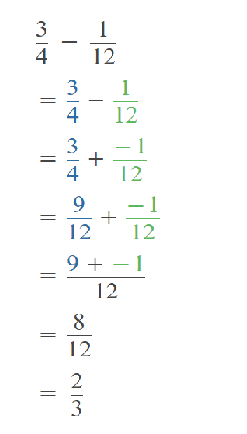Question 8.
$$\frac{3}{5}$$ – $$\frac{3}{9}$$ =
$$\frac{3}{5}$$ – $$\frac{3}{9}$$ = $$\frac{4}{15}$$
Explanation: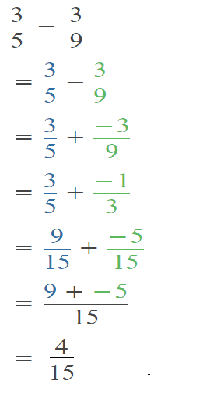Estimate each difference by using the benchmarks, 0, $$\frac{1}{2}$$ or 1. (Lesson 3.2)

Question 9.
$$\frac{4}{5}$$ – $$\frac{3}{8}$$
$$\frac{4}{5}$$ – $$\frac{3}{8}$$ = $$\frac{1}{2}$$
Explanation:
Estimating the fractions to bench marks
$$\frac{4}{5}$$ = 1
$$\frac{3}{8}$$ = $$\frac{1}{2}$$
1 – $$\frac{1}{2}$$  = $$\frac{1}{2}$$

Question 10.
$$\frac{7}{12}$$ – $$\frac{5}{9}$$
$$\frac{7}{12}$$ – $$\frac{5}{9}$$ = 1
Explanation:
Estimating the fractions to bench marks
$$\frac{7}{12}$$  = $$\frac{1}{2}$$
$$\frac{5}{9}$$ = $$\frac{1}{2}$$
$$\frac{1}{2}$$  + $$\frac{1}{2}$$  = 1

Write each fraction as a division expression. (Lesson 3.3)

Question 11.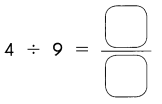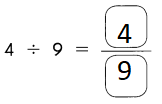Explanation:
$$\frac{4}{9}$$
Fraction into expression.

Question 12.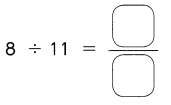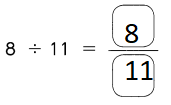Explanation:
$$\frac{8}{11}$$
Fraction into expression.

Write each division expression as a fraction. (Lesson 3.3)

Question 13.
$$\frac{5}{6}$$ = ___ ÷ ___
5 ÷ 6
$$\frac{5}{6}$$ = 5 ÷ 6
Explanation:
converting division expression as a fraction.

Question 14.
$$\frac{7}{12}$$ = ___ ÷ ___
7 ÷ 12
$$\frac{7}{12}$$ = 7 ÷ 12
Explanation:
converting division expression as a fraction.

Complete. (Lesson 3.3)

Question 15.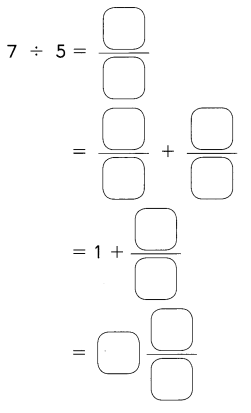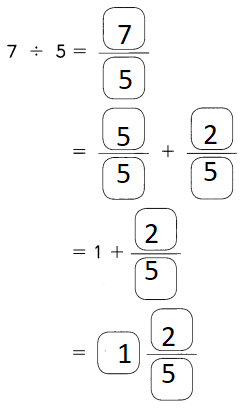Explanation:
Converting the division expression to a mixed fraction

Question 16.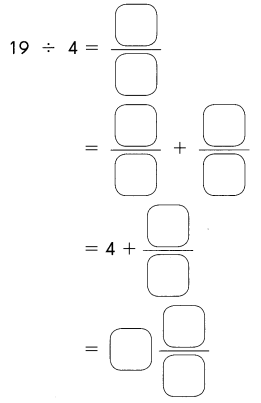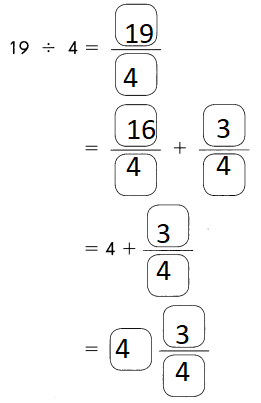Explanation:
Converting the division expression to a mixed fraction

Divide. Express each quotient as a mixed number in simplest form. (Lesson 3.3)

Question 17.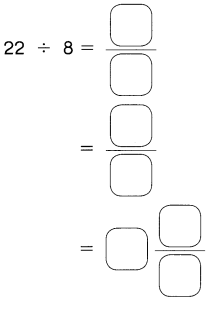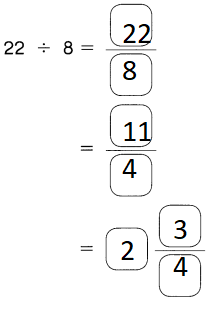Explanation:
Converting the division expression to a mixed fraction

Question 18.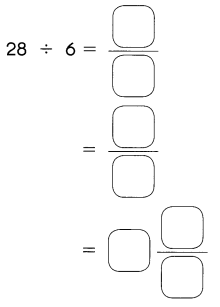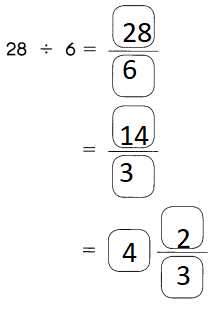Explanation:
Converting the division expression to a mixed fraction

Express each fraction as a decimal. (Lesson 3.4)

Question 19.
$$\frac{4}{5}$$ = ____
= _________
$$\frac{4}{5}$$ = 0.8
Explanation: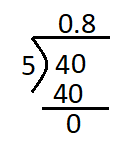Question 20.
$$\frac{17}{20}$$ = ____
= _________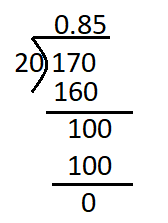Explanation:
$$\frac{17}{20}$$ = 0.85

Express each division expression as a mixed number and as a decimal. (Lessons 3.3 and 3.4)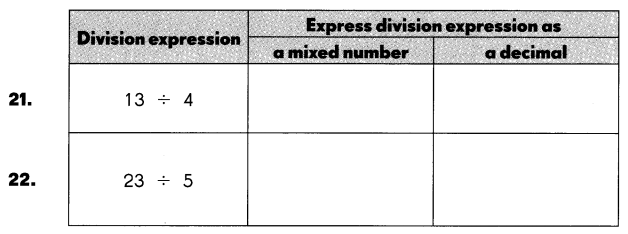Explanation: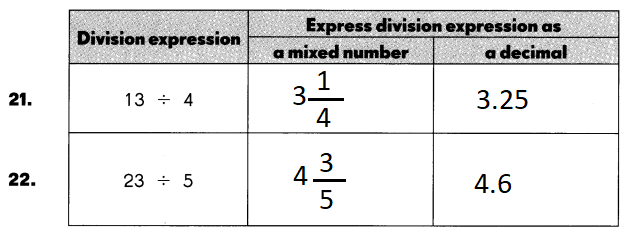Question 21.
13 ÷ 4 = 3$$\frac{1}{4}$$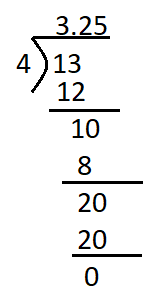Explanation:
13 ÷ 4 = 3.25

Question 22.
23 ÷ 5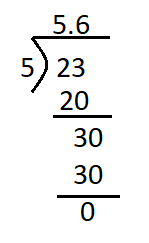Explanation:
23 ÷ 5 = 5.6

Add. Express each sum in simplest form. (Lesson 3.5)

Question 23.
2$$\frac{2}{7}$$ + 3$$\frac{1}{2}$$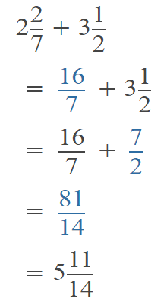2$$\frac{2}{7}$$ + 3$$\frac{1}{2}$$ = 5$$\frac{11}{14}$$

Question 24.
1$$\frac{1}{2}$$ + 1$$\frac{5}{9}$$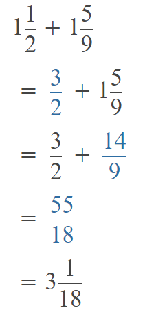Explanation:
1$$\frac{1}{2}$$ + 1$$\frac{5}{9}$$ = 3$$\frac{1}{18}$$

Estimate each sum by using the nearest whole number or half. (Lesson 3.5)

Question 25.
1$$\frac{5}{8}$$ + 1$$\frac{1}{5}$$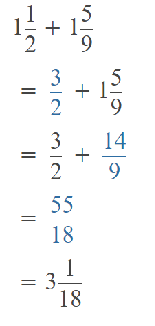Explanation:
1$$\frac{5}{8}$$ + 1$$\frac{1}{5}$$ = 3$$\frac{1}{18}$$ = 3 + 0 = 3
Converting the fraction into a whole number.

Question 26.
2$$\frac{1}{6}$$ + 3$$\frac{4}{5}$$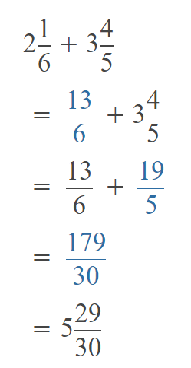Explanation:
2$$\frac{1}{6}$$ + 3$$\frac{4}{5}$$ = 5$$\frac{29}{30}$$
Converting the fraction into a whole number.
5 + 1 = 6

Subtract. Express each difference in simplest form. (Lesson 3.6)

Question 27.
5$$\frac{8}{9}$$ – 3$$\frac{5}{6}$$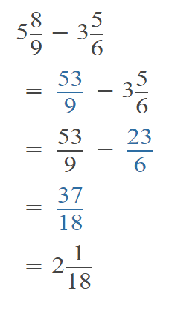Explanation:
5$$\frac{8}{9}$$ – 3$$\frac{5}{6}$$ = 2$$\frac{1}{18}$$
2 + 0 = 2

Question 28.
4$$\frac{2}{7}$$ – 2$$\frac{7}{8}$$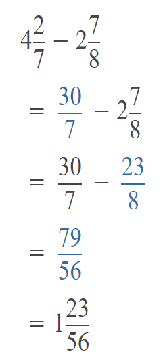Explanation:
4$$\frac{2}{7}$$ – 2$$\frac{7}{8}$$ = 1$$\frac{23}{56}$$
= 1 + $$\frac{1}{2}$$
= $$\frac{3}{2}$$

Estimate difference by using the nearest whole number or half. (Lesson 3.6)

Question 29.
2$$\frac{1}{10}$$ – 1$$\frac{4}{7}$$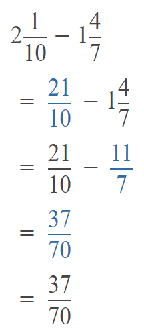Explanation:
2$$\frac{1}{10}$$ – 1$$\frac{4}{7}$$ = $$\frac{37}{70}$$ = $$\frac{1}{2}$$
Converting the fraction into a half

Question 30.
3$$\frac{3}{8}$$ – 1$$\frac{7}{12}$$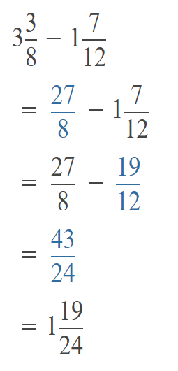Explanation:
3$$\frac{3}{8}$$ – 1$$\frac{7}{12}$$ = 1$$\frac{19}{24}$$
= 1 + 1 = 2

Find the product in simplest form. (Lesson 4.1)

Question 31.
$$\frac{6}{7}$$ × $$\frac{5}{8}$$ =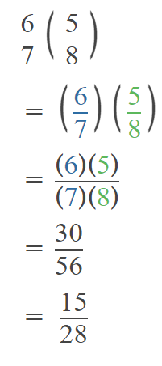Explanation:
$$\frac{6}{7}$$ × $$\frac{5}{8}$$ = $$\frac{15}{28}$$
Multiplication in fractions we have to directly multiply
Numerator x Numerator
and Denominator x Denominator.

Question 32.
$$\frac{4}{5}$$ × $$\frac{10}{12}$$ =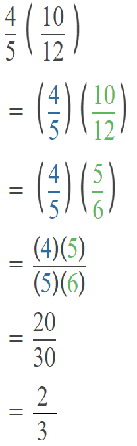Explanation:
$$\frac{4}{5}$$ × $$\frac{10}{12}$$ = $$\frac{2}{3}$$
Multiplication in fractions we have to directly multiply
Numerator x Numerator
and Denominator x Denominator.

Question 33.
$$\frac{2}{5}$$ of $$\frac{10}{11}$$ =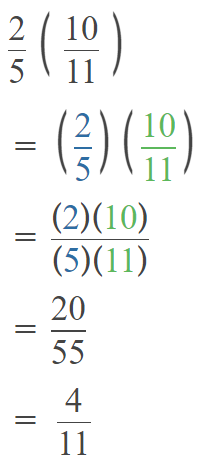Explanation:
$$\frac{2}{5}$$ of $$\frac{10}{11}$$ =$$\frac{4}{11}$$
Multiplication in fractions we have to directly multiply
Numerator x Numerator
and Denominator x Denominator.

Question 34.
$$\frac{8}{9}$$ of $$\frac{5}{12}$$ =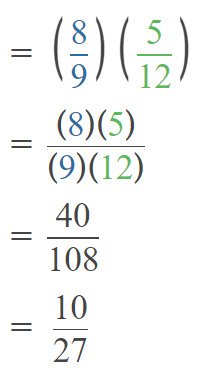Explanation:
$$\frac{8}{9}$$ of $$\frac{5}{12}$$  = $$\frac{10}{27}$$
Multiplication in fractions we have to directly multiply
Numerator x Numerator
and Denominator x Denominator.

Multiply. Express the product in simplest form. (Lesson 4.3)

Question 35.
$$\frac{2}{5}$$ × $$\frac{15}{7}$$ =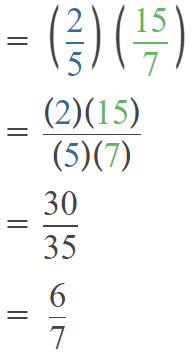Explanation:
$$\frac{2}{5}$$ × $$\frac{15}{7}$$ = $$\frac{6}{7}$$
Multiplication in fractions we have to directly multiply
Numerator x Numerator
and Denominator x Denominator.

Question 36.
$$\frac{9}{5}$$ × $$\frac{5}{12}$$ =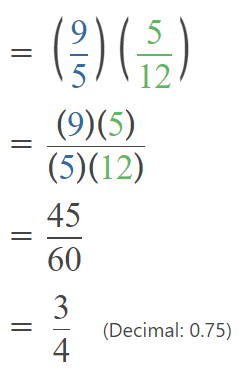Explanation:
$$\frac{9}{5}$$ × $$\frac{5}{12}$$ = $$\frac{3}{4}$$
Multiplication in fractions we have to directly multiply
Numerator x Numerator
and Denominator x Denominator.

Multiply. Express the product as a whole number or a mixed number in simplest form. (Lesson 4.3)

Question 37.
$$\frac{4}{3}$$ × $$\frac{7}{6}$$ =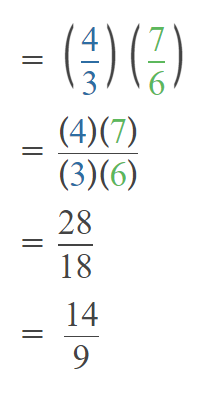Explanation:
$$\frac{4}{3}$$ × $$\frac{7}{6}$$ = $$\frac{14}{9}$$
Multiplication in fractions we have to directly multiply
Numerator x Numerator
and Denominator x Denominator.

Question 38.
$$\frac{8}{3}$$ × $$\frac{9}{12}$$ =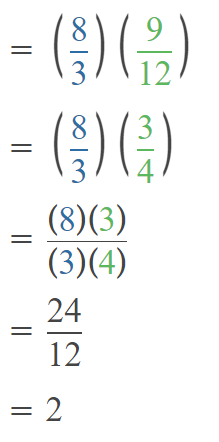Explanation:
$$\frac{8}{3}$$ × $$\frac{9}{12}$$= 2
Multiplication in fractions we have to directly multiply
Numerator x Numerator
and Denominator x Denominator.

Question 39.
$$\frac{7}{8}$$ × $$\frac{6}{5}$$ =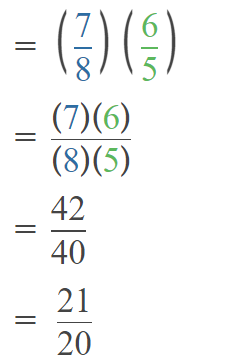Explanation:
$$\frac{7}{8}$$ × $$\frac{6}{5}$$ = $$\frac{21}{20}$$
Multiplication in fractions we have to directly multiply
Numerator x Numerator
and Denominator x Denominator.

Question 40.
$$\frac{25}{4}$$ × $$\frac{10}{8}$$ =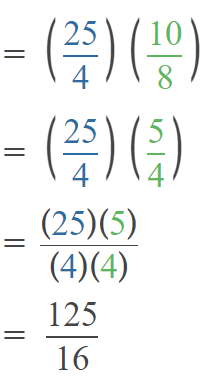Explanation:
$$\frac{25}{4}$$ × $$\frac{10}{8}$$ = $$\frac{125}{16}$$
Multiplication in fractions we have to directly multiply
Numerator x Numerator
and Denominator x Denominator.

Multiply. Express the product as a whole number or a mixed number in simplest form. (Lesson 4.4)

Question 41.
2$$\frac{1}{4}$$ × 16 =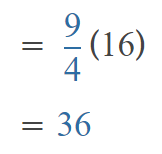Explanation:
Converting the mixed fraction in to a fraction then multiply with 16
And done the simplest form to make into a whole number

Question 42.
27 × 1$$\frac{2}{9}$$ =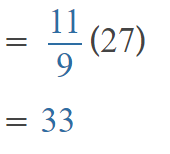Explanation:
Converting the mixed fraction in to a fraction then multiply with 27
And done the simplest form to make into a whole number

Multiply. Express the product as a whole number or a mixed number in simplest form. (Lesson 4.4)

Question 43.
5$$\frac{3}{6}$$ × 42 =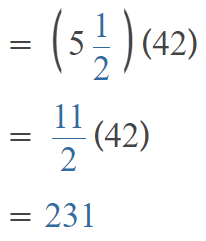Explanation:
Converting the mixed fraction in to a fraction then multiply with 42
And done the simplest form to make into a whole number

Question 44.
2$$\frac{5}{6}$$ × 15 =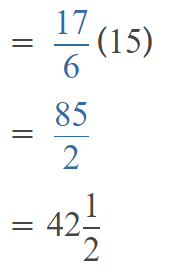Explanation:
Converting the mixed fraction in to a fraction then multiply with 15
And done the simplest form to make into a whole number

Divide. Express each quotient in simplest form. (Lesson 4.6)

Question 45.
3 ÷ $$\frac{1}{9}$$ =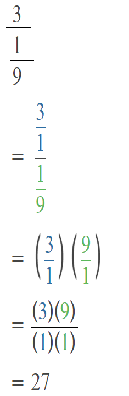Explanation:
Converting the whole number as a fraction
To divide a fraction with a whole number,
we multiply the given whole number with the denominator of the fraction.

Question 46.
6 ÷ $$\frac{1}{8}$$ =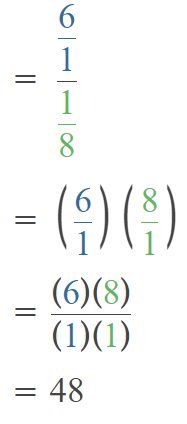Explanation:
Converting the whole number as a fraction
To divide a fraction with a whole number,
we multiply the given whole number with the denominator of the fraction.

Question 47.
5 ÷ $$\frac{1}{5}$$ =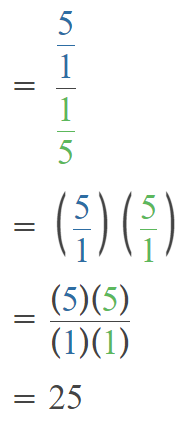Explanation:
Converting the whole number as a fraction
To divide a fraction with a whole number,
we multiply the given whole number with the denominator of the fraction.

Question 48.
2 ÷ $$\frac{1}{10}$$ =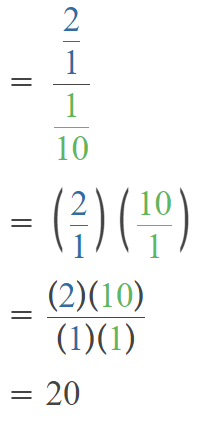Explanation:
Converting the whole number as a fraction
To divide a fraction with a whole number,
we multiply the given whole number with the denominator of the fraction.

Question 49.
$$\frac{7}{8}$$ ÷ 5 =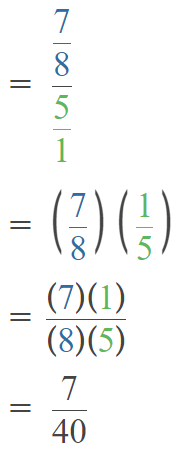Explanation:
Converting the whole number as a fraction
To divide a fraction with a whole number,
we multiply the given whole number with the denominator of the fraction.

Question 50.
$$\frac{5}{8}$$ ÷ 4 =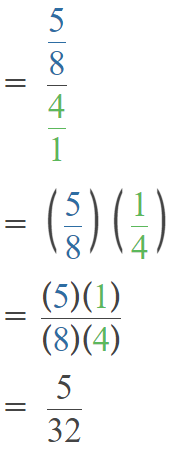Explanation:
Converting the whole number as a fraction
To divide a fraction with a whole number,
we multiply the given whole number with the denominator of the fraction.

Question 51.
$$\frac{4}{7}$$ ÷ 12 =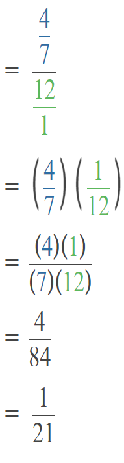Explanation:
Converting the whole number as a fraction
To divide a fraction with a whole number,
we multiply the given whole number with the denominator of the fraction.

Question 52.
$$\frac{2}{9}$$ ÷ 6 =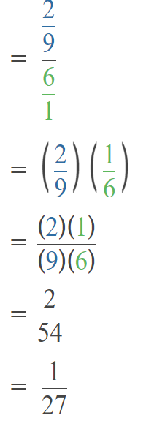Explanation:
Converting the whole number as a fraction
To divide a fraction with a whole number,
we multiply the given whole number with the denominator of the fraction.

Problem Solving

Question 53.
Ron used $$\frac{3}{5}$$ pound of flour to bake bread and $$\frac{2}{7}$$ pound of flour to bake scones. How many more pounds of flour did he use to bake bread than scones?
Answer: $$\frac{11}{35}$$ more pounds of flour he used to bake bread than scones
Explanation:
Subtract them…
But to subtract fractions you need a common denominator (the smallest thing that 5 and 7 both go into)
Remember when making equivalent fractions with your common denominator (which is 7×5 = 35) make sure you multiply the top by whatever you multiplied the bottom by.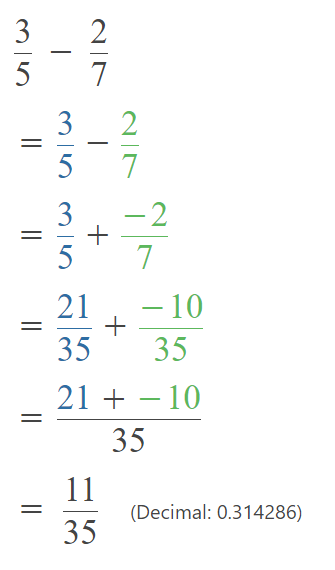Question 54.
Tina uses 4$$\frac{5}{12}$$ yards of wire for her science project. Kelvin uses 1$$\frac{2}{3}$$ yards of wire for his project. How many yards of wire do they use altogether?
Answer: 6$$\frac{1}{12}$$ yards of wire used altogether
Explanation: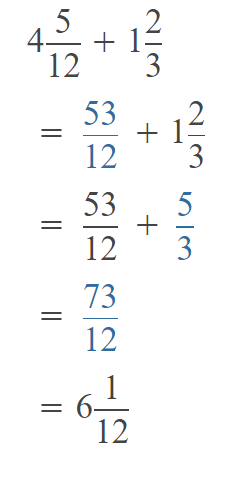Question 55.
Rosa poured 1$$\frac{3}{4}$$ quarts of grape juice into a container. She added 3$$\frac{1}{3}$$ quarts of apple juice. She then poured 2$$\frac{2}{3}$$ quarts of the mixed juice into a pitcher. How many quarts of mixed juice were left in the container?
Answer: 2$$\frac{5}{12}$$
Explanation:
1$$\frac{3}{4}$$ =1$$\frac{9}{12}$$
3$$\frac{1}{3}$$ = 3$$\frac{4}{12}$$

1$$\frac{9}{12}$$+ 3$$\frac{4}{12}$$ = 4$$\frac{13}{12}$$ = 5$$\frac{1}{12}$$

4$$\frac{13}{12}$$ – 2$$\frac{8}{12}$$ = 2$$\frac{5}{12}$$

Question 56.
A race was $$\frac{11}{12}$$ mile long. Hamish ran $$\frac{4}{5}$$ of the distance.

a. Without multiplying, explain how you know that the answer must be less than $$\frac{11}{12}$$.
With the help of bench mark. Both the values are lesser than 1. So he ran less than $$\frac{11}{12}$$.

b. How far did he run?
Answer: $$\frac{7}{60}$$
Explanation: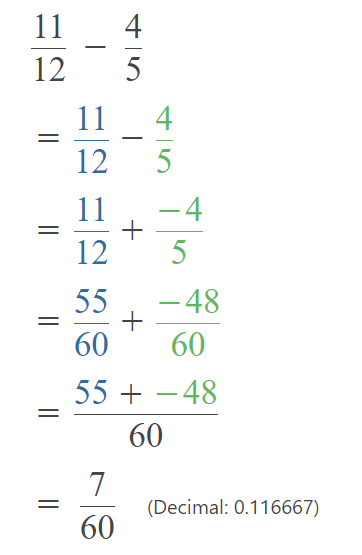Question 57.
Ashley uses $$\frac{1}{4}$$ package of raisins for o fruit cake. She then uses $$\frac{1}{9}$$ of the remainder for muffins. What fraction of the package of raisins does she have left?
Answer: $$\frac{23}{36}$$ of the package of raisins she have left
Explanation: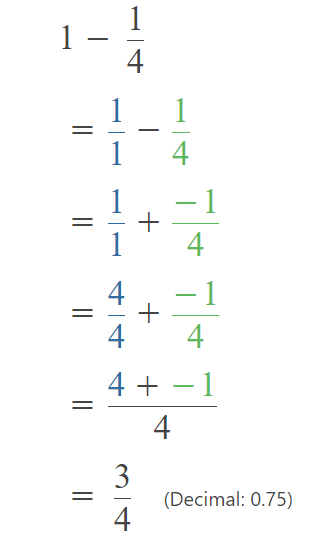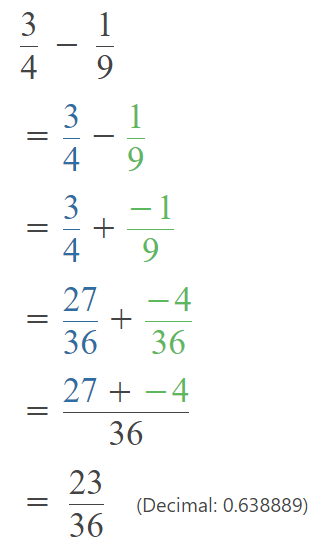Question 58.
Mrs. Vernon used 4$$\frac{3}{8}$$ pounds of meat in each of her 12 pots of soup. How many pounds of meat did she use for the 12 pots of soup?
Answer: 52$$\frac{1}{2}$$ pounds of meat she used.
Explanation: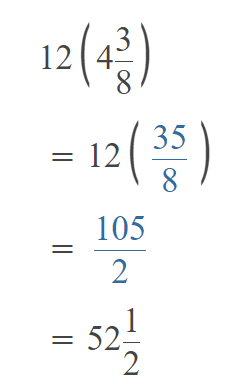Question 59.
A custodian pours $$\frac{1}{8}$$ gallon of cleaning solution into each pail of water that she uses.

a. How many pails of water and cleaning solution can the custodian make using 16 gallons of cleaning solution?

b. Find the volume of solution in two of these pails.

Question 60.
A carnival sold 135 bottles of juice in one day. They sold $$\frac{1}{3}$$ of the bottles in the first hour and $$\frac{2}{5}$$ of the bottles in the second hour. How many bottles of juice did they sell altogether in these two hours?
Answer: 99 bottles sold altogether in these two hours
Explanation: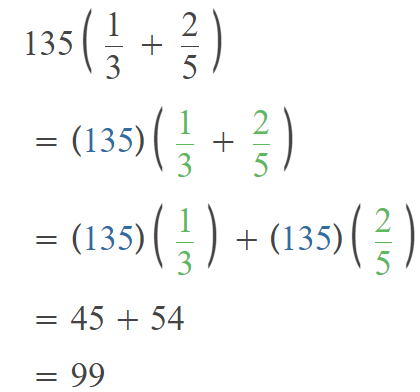Question 61.
Ms. Li spent $840 on a vacation. She spent $$\frac{2}{3}$$ of the amount on a plane ticket and $$\frac{1}{2}$$ of the remaining amount on food. How much did she spend on the ticket and food altogether? Answer: So she spent$560+$140 =$ 700  on the ticket and food altogether
Explanation:
Ms. Li spent $840 on a vacation. She spent $$\frac{2}{3}$$ of the amount on a plane ticket. So she spent$560 for plane ticket.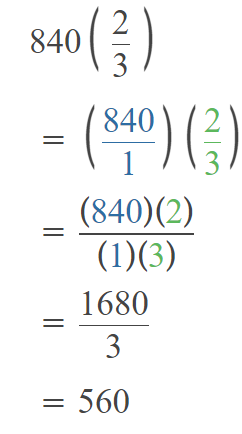She spent $$\frac{1}{2}$$ of the remaining amount on food.
Remaining amount is $280. So she spent$ 140 on food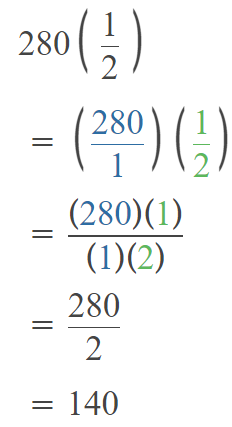So she spent $560+$140 = \$ 700  on the ticket and food altogether

Question 62.
Sam traveled $$\frac{3}{4}$$ of a journey by bus. He jogged $$\frac{1}{2}$$ the remaining distance and walked the rest of the way. If he walked 800 feet, what was the total distance he traveled?
Explanation:
Total Distance = x
Sam traveled $$\frac{3}{4}$$ of a journey by bus. So it is $$\frac{3}{4}$$ x
So remaining distance is x – $$\frac{3}{4}$$ x =$$\frac{1}{4}$$ x
He jogged $$\frac{1}{2}$$ the remaining distance. So he jogged $$\frac{1}{8}$$ x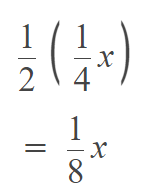walked the rest of the way . So remining is x – $$\frac{3}{4}$$ x + $$\frac{1}{8}$$ x  =800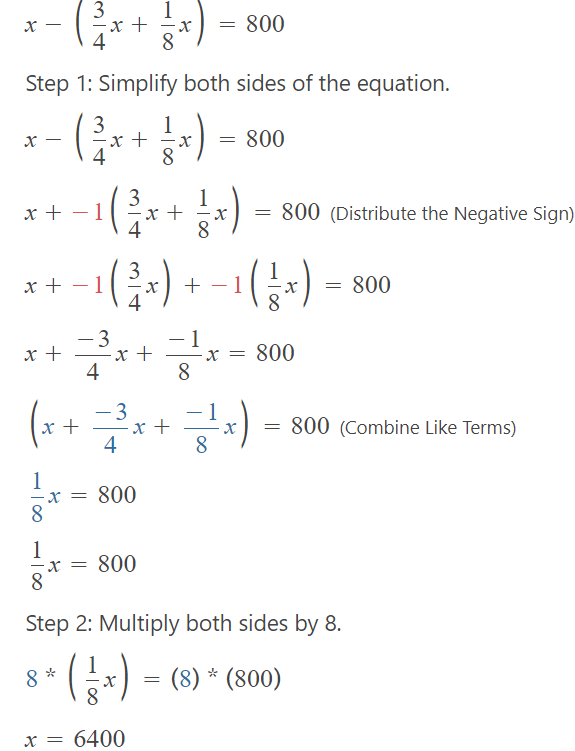Question 63.
Matthew used $$\frac{1}{5}$$ of a box of flour for cooking and $$\frac{3}{4}$$ of the remainder to make bread. The rest of the flour was packed equally into 5 containers. What fraction of the total amount of flour was in each container? r
Answer: $$\frac{1}{100}$$ fraction of the total amount of flour was in each container
Explanation:
Matthew used $$\frac{1}{5}$$ of a box of flour for cooking. So remaining flour is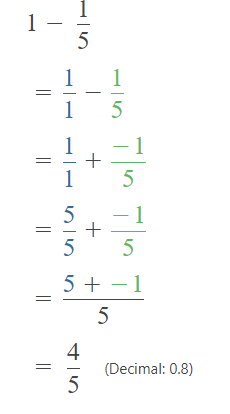$$\frac{3}{4}$$ of the remainder to make bread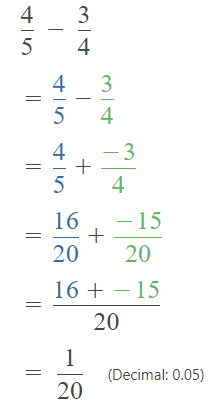The rest of the flour was packed equally into 5 containers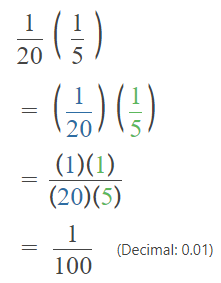Question 64.
A bus driver filled up $$\frac{7}{8}$$ of her fuel tank for a trip. She used $$\frac{6}{7}$$ of the fuel by the end of the trip. The capacity of her tank is 70 gallons. How much fuel did she use for the trip? Express your answer as a decimal.
Answer: 52.5gallons of fuel she used for the trip
Explanation:
The capacity of her tank is 70 gallons.
A bus driver filled up $$\frac{7}{8}$$ of her fuel tank for a trip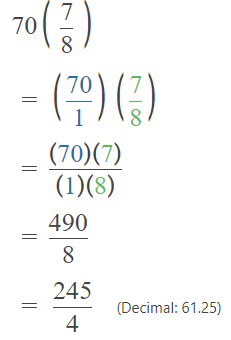So she filled 61.25 gallons of fuel in tank.
She used $$\frac{6}{7}$$ of the fuel by the end of the trip.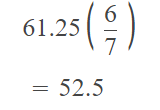Question 65.
Sergio walked to and from school on $$\frac{3}{5}$$ of the days in one month. He got a ride to school on $$\frac{7}{8}$$ of the remaining days. On the one remaining school day that month, he stayed home with a cold. How many school days were there that month?
Sergio walked to and from school on $$\frac{3}{5}$$ of the days in one month = $$\frac{3}{5}$$x
So remaining days = x – $$\frac{3}{5}$$x = $$\frac{2}{5}$$x
He got a ride to school on $$\frac{7}{8}$$ of the remaining days. = $$\frac{7}{20}$$x .Since